Courses

# CAT Quantitative Aptitude MCQ - 2

## 34 Questions MCQ Test CAT Mock Test Series 2020 | CAT Quantitative Aptitude MCQ - 2

Description
This mock test of CAT Quantitative Aptitude MCQ - 2 for CAT helps you for every CAT entrance exam. This contains 34 Multiple Choice Questions for CAT CAT Quantitative Aptitude MCQ - 2 (mcq) to study with solutions a complete question bank. The solved questions answers in this CAT Quantitative Aptitude MCQ - 2 quiz give you a good mix of easy questions and tough questions. CAT students definitely take this CAT Quantitative Aptitude MCQ - 2 exercise for a better result in the exam. You can find other CAT Quantitative Aptitude MCQ - 2 extra questions, long questions & short questions for CAT on EduRev as well by searching above.
QUESTION: 1

### 5 men and 6 women are to be arranged in a straight line for a photograph. In how many ways this can be done such that two particular women Sheela and Chameli are always together and no two men are beside each other?

Solution:

Since two women are always together, 6 women can be arranged in 5! x 2! = 240 ways.
Now, 5 men can stand at 6 different gaps created between the women.
They can be arranged in 6P5 = 6! = 720 ways

So, total number of ways = 240 x 720 = 172800

Hence, option 2.

QUESTION: 2

### What is the highest power of 48 in 32!?

Solution:

Because factorisation of 48 is  24x3 , so highest power of 2 will be 31 and 3 will be 14 so answer should be 14

*Answer can only contain numeric values
QUESTION: 3

### What will be the number of zeroes at the end of the product of the first 40 natural numbers?

Solution:

Number of zeroes in a number can be found by calculating the highest power of 10.

10 = 2 x 5

Number of zeroes will be highest power of 5 in 40!.
Highest power of 5 in 40!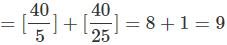QUESTION: 4

I reached office 5 minutes late while walking at a speed of 5 m/sec. But I could have reached 3 minutes before time by walking at 5 times my original speed. Find the distance that I have to walk daily (in km).

Solution:

Let V be the distance that I have to walk daily to reach office.
Let the original time required be t .
Let the orginal speed required be v.
5 minutes late (300 seconds):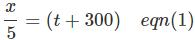3 minutes early (180 seconds)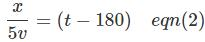Orginal time required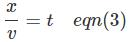From eqn(2) and eqn(3),we get
t = 225 seconds
Put the value of t in eqn(1),
we get, x = 2625 m i.e 2.63 km
Hence, option 2.

QUESTION: 5

The total number of relations from set P to set Q are 4096. If x and y are the number of elements in sets P and Q respectively, find the number of values the ordered pair (x, y) can take.

Solution:

Let us define a function from a set P of 3 elements (a, b, c) and a set Q with 4 elements (w, x, y, z). Now, for a function to be defined from set P to set Q, every element of P must connect with exactly one element of Q while every element of Q can connect to multiple elements of P.
Thus, the three elements of set P each has four options from Q. Thus, the total number of relations in this case = 43 = 64.
Now, the number of relations given is 4096. xy = 4096

(x, y) = (4096, 1); (2, 12); (4, 6); (8, 4); (16, 3); (64, 2)

Hence, there are 6 such pairs possible.
Hence, option 4.

*Answer can only contain numeric values
QUESTION: 6

The annual income of X and Y is in the ratio 6 : 5 and their expenses are in the ratio 9 : 7. If each saves Rs. 3000 at the end of the year, find the sum of their annual incomes.

Solution:

Let the income of X and Y be 6x and 5x respectively.
Let he expenses of X and Y be 9y and ly respectively.

6x- 9y = 3000 ... (1)

5x- 7y = 3000 ... (2)

By solving these two equations we get,

42x - 45x = -6000

x = 2000

Therefore, income of x = 2000 x 6 = 12000 And income of Y = 2000 x 5 = 10000

Sum of their incomes = 12000 + 10000 = 22000

QUESTION: 7

The sum of three numbers is zero and the the product of the three numbers is -120, find the sum of the cubes of the three numbers.

Solution:

If a + b + c = 0, a* + 63 + c3 = 3 abc

The sum of the cubes of three numbers = -120 x 3 = -360

Hence, option 3.

QUESTION: 8

The per month average salary of the employees of a firm is Rs. 20,000. All the employees are given a raise of 15% after the appraisal month. However, some people resigned from the firm and the funds allocated towards giving away their salary was distributed amongst all. Resultantly, the average rise in the salary of the currently working employees increased by Rs. 2,000. If 46 employees are currently working with the company then find the number of employees who resigned.

Solution:

Let the number of employees who left be x.
The total funds allocated towards the salary after increment = (46 + x) x 20000 x 1.15

The new salary per employee = 20000 x 1.15 + 2000 = 25000

(46 + x) x 20000 x 1.15 = 25000 x 46 Solving for x, we get

x = 4

Hence, option 1.

QUESTION: 9

The 3rd term of an A.R is 23 and 8th term is 58. Find the 80th term.

Solution:

Let the 1st term (t1) of an A.R be a and common difference be d.

t3 = a + 2d = 23 ... (i)

Similarly,

t8 = a + 7d = 58 ... (ii)

Solving these equation we get,

a = 9 and d = 7

t8= a + 79d = 9 + 79(7) = 562

Hence, option 2.

QUESTION: 10

On planet K-Pax, the dial of a clock has 14 equally spaced markings. The day is divided into 28 hours; each hour is divided into 70 minutes and each minute is divided into 70 seconds. Find that number of times that the hour hand and minute are exactly opposite to each other in a day.

Solution:

Consider the clock at 14 AM in the night.

The hour hand and minute hand are opposite to each other once before the time is 1 AM. They are again opposite to each other once before the time is 2 AM. And so on in every hour till 7 AM.

Between 6 AM and 8 AM, the hands are opposite to each other only once, at 7 AM. Again the hands are opposite to each other once every hour from 9 AM to 14 noon.

So, in a span of 14 hours, the number of times that the two hands are opposite to each other is 13.

So, in 28 hours, the number of times that the two hands are opposite to each other is 13*2 = 26

QUESTION: 11

What is the value of x + √x, if log2 X + log2 X2 + log2 X3 +...+ log2 X10= 440?

Solution:

The equation can be written as, (1 + 2 + 3 + ...+ 10)log2x = 440
(11 x 10/2)log2x = 440
log2X = 8
x = 28 = 256
Thus, x = 256 and √x = 16
∴ x + √x = 272
Hence, option 3.

QUESTION: 12

A train 125 m long passes a man, running at 5 km/hr in the same direction in which the train is going, in 10 seconds. The speed of the train is:

Solution: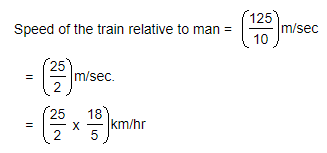= 45 km/hr.

Let the speed of the train be x km/hr. Then, relative speed = (x - 5) km/hr.x - 5 = 45x = 50 km/hr.

QUESTION: 13

The ratio of the sum to product of two digits of a two-digit number is 2 : 3. If one of the digits exceeds the other by 4, then find the number of highest value which can be formed using the two digits.

Solution:

Let one of the digits be x.
∴ The other digit will be x + 4
Now,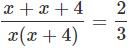6x + 12 = 2x2 + 8x
x2 + x - 6 = 0
x = 2, -3
Since, -3 cannot be one of the digits the digits are 2 and 6.
Thus, 62 is the number with highest value which can be formed using the two digits.
Hence, option 2.

QUESTION: 14

What will be the co-ordinates of the foot of the perpendicular drawn from the point (3, 4) to the line 4x + 2y - 9 = 0?

Solution:

Let (a, b) is the required co-ordinates of the foot of the perpendicular.

4a + 2b - 9 = 0 ... (i)

Slope of the above line = -2

Slope of the line perpendicular to the above line =1/2 (b - 4)/(a - 3) = 1/2

a-2b = —5 ... (ii)

Solving the equations (i) and (ii) we get,

a = 4/5 and b = 29/10

Hence, option 2.

QUESTION: 15

The roots of the quadratic equation x2 + bx + c = 0, having distinct roots, are non-zero and integral solutions of the inequality, y2 + 2y - 3 < 0. What is the value of b + c?

Solution:

y2 + 2y - 3 = (y - l)(y + 3) < 0

-3 < y < 1

y can take the integral values -2, -1, 0

Roots o f the given equation can be - 2 , - 1 , 0

As roots are non-zero, therefore, the roots of the equation x2 + bx + c = 0 are -2,-1.
x2 + bx + c = (x + 2)(x + 1) = 0

x2 + bx + c = x2 + 3x + 2 = 0

b = 3 and c = 2

b + c = 5

Hence, option 2.

*Answer can only contain numeric values
QUESTION: 16

A family spends Rs. 3,000 per month to purchase 15 kg grocery. If the rate per kg of the grocery increases by 20%, how much less grocery (in kg) should the family purchase to keep the expenditure same?

Solution:

Original per kg cost of grocery = 3000/15 = Rs. 200

New rate = 1.2 x 200 = Rs. 240

Grocery purchased after increase in price = 3000/240 =12.5kg

Reduction in consumption = 15 - 12.5 = 2.5 kg

QUESTION: 17

What is the diagonal of a square (in cm) having its area equal to that of a rhombus having its diagonals as 12 cm and 14 cm?

Solution:

Area of rhombus = (1/2) x 12 x 14 = 84 cm2 Let the diagonal of the square be d

(1/2) x d2 = 84

Diagonal of the square = d = 2√42 cm Hence, option 1.

*Answer can only contain numeric values
QUESTION: 18

A train 440 metres long passes a railway platform 876 metres long in 10 seconds. How many seconds will it take to pass a running train 990 metres long? (Enter 0 if the answer cannot be determined.)

Solution:

As we do not know in which direction the other train is running, we cannot determine answer.

QUESTION: 19

Find the selling price of a sari after successive discounts of 20%, 25% and 30% on the marked price of Rs. 700.

Solution:

Selling price after successive discount = 700 x 0.8 x 0.75 x 0.7 = Rs. 294

Hence, option 1.

QUESTION: 20

Two circles with different radii touch each other in one point such that the smaller circle lies completely inside the larger circle. The smaller circle has point O as the center. A chord AB of the larger circle is tangent to the smaller circle. AB touches smaller circle at point C. A and B lies on the circumference of the larger circle. If BC = 10 cm and OB = 20 cm. Find OC (in cm).

Solution:

AB touches smaller circle at C. So, OC ⊥ AB.
Now, in AOCB, OB = 20 cm and BC =10 cm. By pythagoras theorem we get, OC = 10√3 cm
Hence, option 1.

*Answer can only contain numeric values
QUESTION: 21

The average salary per employee in an office is Rs. 3,200 per month. The average salary of officers is Rs. 6,800 and that of non-officers is Rs. 2,000. If the number of officers is 5, then find the number of non-officers in the office.

Solution:

Let the number of officers and non-officers be n and m respectively.

Total salary of officers = 6800n And, Total salary of non-officers = 2000m

Total salary of all the employees = 6800n + 2000m = 3200(m + n)

3600n = 1200m

3n = m

m = 3 x 5 = 15

Number of non-officers =15

QUESTION: 22

In a set of 17 points, exactly 5 points are collinear. How many triangles can be formed using these points? (Assume all points are distinct)

Solution:

We need to select exactly three points from the given 17 points to form a triangle.
However, no triangle can be formed if all the three points selected are collinear.
Number o f triangles formed = 17C3 - 5C3 = 670

Hence, option 4.

QUESTION: 23

The perimeter of an isosceles triangle is equal to 14 cm and the ratio of the lateral side and the base is 5 : 4. Find the area of the triangle (in cm2)

Solution:

Let 5x be the lateral side of the triangle and 4x be the base of the triangle.
5x + 5x + 4x= 14
x =1
On solving the two equation we get y = 4 and x = 5.
We get the following triangle.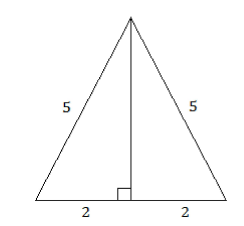By Pythagoras theorem,
Height of the triangle = √52 - 22 = √21 cm
Hence, area of the triangle = 1/2 x 4 x √21 = 2√21 cm2
Hence, option 4.

QUESTION: 24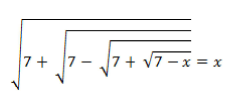Which of the following can be value of x?

Solution:

On substituting the values from the options given above, we see that x = 3 is the only value that satisfies the given equation.
Hence, option 2.

*Answer can only contain numeric values
QUESTION: 25

If 'f' is the real function satisfying 2f(xy) = [f(x)]y + [f(y)]x for all real values of x, y and f(1) = 2, then f(1) +f(2)+f(3) + ... +f(10) is equal to

Solution:

2f[xy) = [f(x)]y + [f(y)]x for all real x, y Replacing ‘y’ by 1, we get,
∴ 2 x f(x) = [f(x)]+ [f(1)]x
∴ f(x) = 2x
∴ f(2) = 22 = 4,f(3) = 23 = 8 and so on.
∴ f(1) +f(2) + ... + f(10) = 21 + 22 + 23 + ... + 210
The above series is in G.R with ratio = 2 and initial term = 2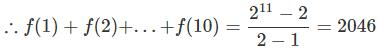QUESTION: 26

If 343x+2 - 49x+3 + 7x+4 = 0, which of these could be a value for x?

Solution:

343x+2 - 49x+3 + 7x+4 = 73x + 6 - 72x+6 + 7x+4
∴ 7x+4(72x +2 - 7x+2 + 1) = 0
Now, 7x+4 ≠ 10
∴ 72x + 2 - 7x+2 + 1 = 0
Let 7x+1 = y
∴ y - 7y + 1 = 0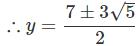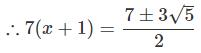Taking log7 on both sides,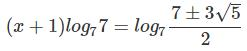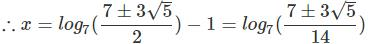Hence, option 3.

QUESTION: 27

The length of the common tangent of two circles of different radius is 15 cm and it divides the line joining the centre of the two circles in the ratio 2 : 1. Find the area of the larger circle if the length of the line joining the centre of two circles is 39 cm.

Solution: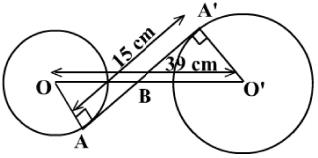Consider the given diagram.
∠ OBA ≌ ∠O'BA'... (Vertically opposite angles)
∠ OAB ≌ Z.O'A'B ... (Radius is perpendicular to the tangent)
∴ ΔOAB ~ AO'A'B ... (AA test of similarity)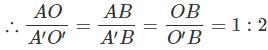Given that OO ' = 39 cm and AA' = 15 cm

O'B = 26 cm and A'B = 10 cm

O'A' = (O'B2 - A'B2)1/2 = 24 cm

Area of the larger circle = 576π

Hence, option 2.

QUESTION: 28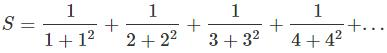What is the value of S?

Solution: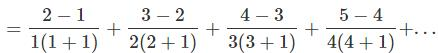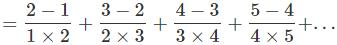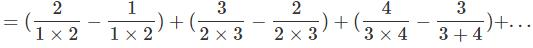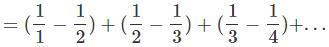= 1
Hence, option 3.

*Answer can only contain numeric values
QUESTION: 29

Sushil is standing 25 m away from a tower. If the angle of elevation of the tower from this point is 45°, find the height (in m) of the tower.

Solution:

The angle of elevation is 45°.
So, height of the tower will be same as the distance of the tower from Sushil.
The height of the tower is 25 m

QUESTION: 30

X alone takes 5 days and Y alone takes 7 days to finish a job. How many days will X and Y take to finish the job working on alternate days starting with X?

Solution:

Let the total work be LCM of number of days taken by X and Y individually.

Total work = LCM (5, 7) = 35 unit

In 2 days, X & Y will complete (12/35)th of the total work.
In this manner they will complete (24/35)th work in 4 days.
After this X will work for 1 day and he completes 1/5th work.
After 5th day, work left = (11/35 - 1/5) = 4/35 On 6th day, Y will do the remaining 4/35th work in 4/5th of days time.
So total time taken is 5 + 4/5 days.
Hence, option 4.

QUESTION: 31

In a geometric progression, there is exactly one term between the terms 24 and 3456. What will be the ratio of the 4th term to the 2nd term of the geometric progression?

Solution:

Let r be the common ratio of the geometric progression.
The fourth term of the geometric progression, t4 =t2 x r2, where t2 is the second term of the geometric progression.

t:t2 = r:1

Also, 3456 = 24 x r2 => r2 = 144

t4 : t2 = 144 : 1

Hence, option 4.

QUESTION: 32

Some quantity of juice from a container of 60 litres of apple juice is replaced with mango juice. Same quantity of the mixture is drawn again and is replaced by equal quantity of mango juice. After this, quantity of apple juice left in the container is (4/9)th of the initial quantity. What quantity of the liquid is taken out from the container both time (in litres)?

Solution:

Let the qunatity drawn from the container both times be x.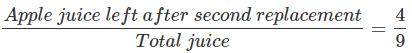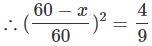Solving this we get, x = 20 litres
Hence, option 2.

*Answer can only contain numeric values
QUESTION: 33

What is the number of real roots of the equation px2 + qx| + r = 0, where p, q and r are positive real numbers?

Solution:

px2 + q|x|+ r = 0

p , q and r are positive, x2 and x are also non-negative. The sum o f nonnegative and positive numbers cannot be zero.

The equation has no real roots.

*Answer can only contain numeric values
QUESTION: 34

In the fig., ST = 6 cm, QR = 10 cm and PS = 9 cm. Find PR (in cm).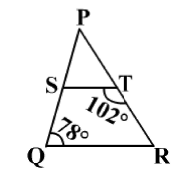Solution:

In the ΔPQR and ΔPTS,

m∠QPR = m∠TPS ... (Same angles with different names)

m∠PTS + m∠STR = 180°

m∠PTS = 180° - m∠STR = 180° - 102° = 78°

Also, m∠PQR = 78°

m∠PQR = m∠PTS

ΔPQR ~ ΔPTS
PR / PS = QR / TS
PR = QR x PS / TS = 10 x 9 / 6 = 15